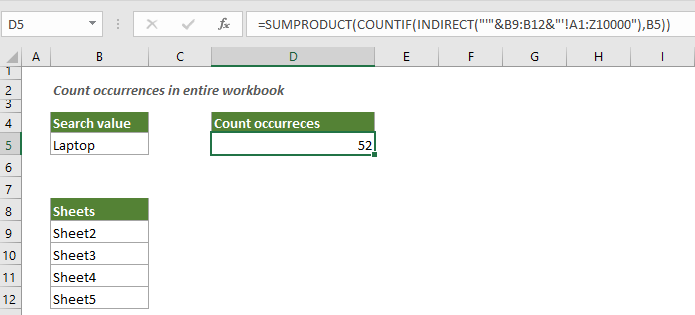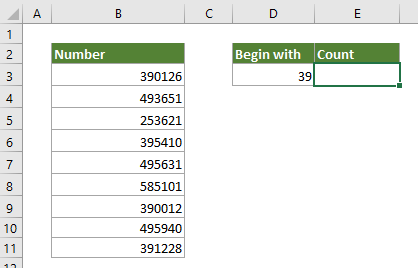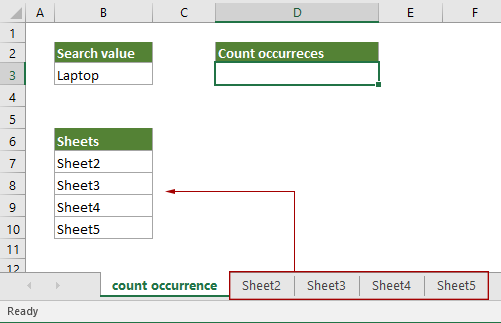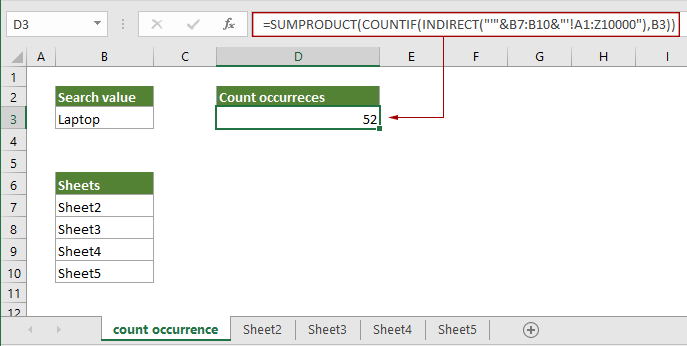## Count occurrences of particular text in entire Excel workbook

It is easy for us to count how many times particular text or a number value occurs in a range of cells with the COUNTIF function. However, to count occurrences of particular text in entire workbook, a more complex formula is required. This article is going to demonstrate a formula based on the SUMPRODUCT, COUNTIF and INDIRECT function to solve this problem.#### How to count occurrences in entire workbook?

Supposing there are 4 worksheets (Sheet2, Sheet3, Sheet4 and Sheet5) in your workbook, and you want to count how many times the particular text “Laptop” appears across these 4 worksheets, you can do as follows to get it done.Generic Formula

=SUMPRODUCT(COUNTIF(INDIRECT(“‘”&range1&”‘!”&range2),criteria))

Arguments

Range1: The range of cells lists all sheet names of current workbook.
Range2: The range of cells you want to count the specific value from.
Criteria: The particular text you will count across sheets.

How to use this formula?

1. You will first need to create a new sheet and list all sheet names in a range of cells. In this case, I list all sheet names in range B7:B10.2. Select a blank cell to output the result.

3. Enter one of the below formulas into it and press the Enter key to get the result.

If you have already entered the particular text into a cell (such as B3), you can reference this cell in the formula as follows:

=SUMPRODUCT(COUNTIF(INDIRECT("'"&B7:B10&"'!A1:Z10000"),B3))

Or directly type the particular text enclosing with double quotation marks in the formula like this:

=SUMPRODUCT(COUNTIF(INDIRECT("'"&B7:B10&"'!A1:Z10000"),"Laptop"))Note: Here the range A1:Z10000 is an arbitrary range, you can change it to suit your data

How this formula works?

=SUMPRODUCT(COUNTIF(INDIRECT("'"&B7:B10&"'!A1:Z10000"),B3))

• "'"&B7:B10&"'!A1:Z10000": Here each sheet name in the array joined via concatenation to the range A1:Z10000, and you will get an array as {"'Sheet2'!A1:Z10000";"'Sheet3'!A1:Z10000";"'Sheet4'!A1:Z10000";"'Sheet5'!A1:Z10000"}.
• INDIRECT({"'Sheet2'!A1:Z10000";"'Sheet3'!A1:Z10000";"'Sheet4'!A1:Z10000";"'Sheet5'!A1:Z10000"}): The INDIRECT function refers the ranges in the four sheets.
• COUNTIF(INDIRECT("'"&B7:B10&"'!A1:Z10000"),B3): The COUNTIF function counts the number of times the specific value appears in each sheet and returns an array {13;13;13;13}. It means that the particular text appears 13 times in each sheet.
• SUMPRODUCT({13;13;13;13}): The SUMPRODUCT function sums all numbers in the array and returns the final result as 52.

#### Related functions

Excel SUMPRODUCT function
The Excel SUMPRODUCT function can be used to multiply two or more columns or arrays together, and then get the sum of products.

Excel COUNTIF function
The Excel COUNTIF function is a statistical function in Excel which is used to count the number of cells that meet a criterion.

Excel INDIRECT function
The Excel INDIRECT function converts a text string to a valid reference.

#### Related formulas

Count numbers that begin with a specific number
This tutorial provides a formula based on the SUMPRODUCT and LEFT functions to count number of cells containing numbers that begin with a specific number(s) in Excel.

Count multiple criteria with NOT logic in Excel

Count or sum only whole numbers in Excel
This post provides two formulas based on the SUMPRODUCT function and the MOD function to help you count and sum only whole numbers in a range of cells in Excel.

Count numbers where the nth digit equals to a given number
This tutorial provides a formula based on the SUMPRODUCT and the MID function to count numbers where the nth digit equals to a given number in Excel.

### The Best Office Productivity Tools

#### Kutools for Excel - Helps You To Stand Out From Crowd

 Popular Features: Find, Highlight or Identify Duplicates  |  Delete Blank Rows  |  Combine Columns or Cells without Losing Data  |  Round without Formula ... Super VLookup: Multiple Criteria  |  Multiple Value  |  Across Multi-Sheets  |  Fuzzy Lookup... Adv. Drop-down List: Easy Drop Down List  |  Dependent Drop Down List  |  Multi-select Drop Down List... Column Manager: Add a Specific Number of Columns  |  Move Columns  |  Toggle Visibility Status of Hidden Columns  |  Compare Columns to Select Same & Different Cells ... Featured Features: Grid Focus  |  Design View  |  Big Formula Bar  |  Workbook & Sheet Manager | Resource Library (Auto Text)  |  Date Picker  |  Combine Worksheets  |  Encrypt/Decrypt Cells  |  Send Emails by List  |  Super Filter  |  Special Filter (filter bold/italic/strikethrough...) ... Top 15 Toolsets:  12 Text Tools (Add Text, Remove Characters ...)  |  50+ Chart Types (Gantt Chart ...)  |  40+ Practical Formulas (Calculate age based on birthday ...)  |  19 Insertion Tools (Insert QR Code, Insert Picture from Path ...)  |  12 Conversion Tools (Numbers to Words, Currency Conversion ...)  |  7 Merge & Split Tools (Advanced Combine Rows, Split Excel Cells ...)  |  ... and more

Kutools for Excel Boasts Over 300 Features, Ensuring That What You Need is Just A Click Away...#### Office Tab - Enable Tabbed Reading and Editing in Microsoft Office (include Excel)

• One second to switch between dozens of open documents!
• Reduce hundreds of mouse clicks for you every day, say goodbye to mouse hand.
• Increases your productivity by 50% when viewing and editing multiple documents.
• Brings Efficient Tabs to Office (include Excel), Just Like Chrome, Edge and Firefox.